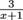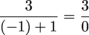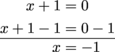Study Guide

# Basic Algebra - Dividing by Zero

## Dividing by Zero

Let's review a math rule. Remember this one? No dividing by zero. Nuh-uh, no way, never, ever. Don't do it. Ever wonder why?

A division problem can be read as a multiplication problem that's missing a number. "What is 24 divided by 6?" is the same question as "6 times what is 24?" Both answers are 4.

Let's try that with 0. The division problem "what is 24 divided by 0?" is the same as the question "0 times what is 24?" And boom, there it is—impossible, no answer, can't be done, nuh-uh, no way, never ever. There is nothing we can multiply 0 by to get 24. In fact, there is nothing we can multiply 0 by to get any number (other than 0 itself), because 0 times anything is just 0. A number divided by 0 is like matter getting sucked into a black hole: it becomes undefined.

This little fact explains a lot of messes in the world of Algebra. For example, the simple little expressionis easy-peasy if x = 2 or 81 or -2347, but if x = 0, it melts into a puddle and evaporates into nothing.

So now that we have variables (letters that supposedly can be any number) and fractions (two numbers being divided), we need to make sure no zeros sneak into our denominators. If a fraction does have 0 in the denominator, it's undefined.

### Sample Problem

What values of x will makeundefined?

When x = 0, then the denominator will equal 0. That'll make the fraction undefined.

### Sample Problem

What values of x will makeundefined?

This is a little trickier, but not much. The question is, what would x need to be to make x + 1 = 0? If we remember our integers, we know that -1 + 1 = 0. If x = -1, then we get a denominator of 0 and an undefined expression. Check it:Basically, all we're doing is setting the denominator equal to 0 and then solving for x.Here's the trick, though. We've solved for the number we don't want x to be, ever. Every other time we solve for x we're solving for the number x is, not the number it can't be. But when we're trying to find where the expression is undefined, we're trying to find what we don't want x to be (unless we're trying to make a black hole).

Though we will say, it's pretty funky what getting close to 0 in a denominator does to the graphs of equations. But that's for another day.